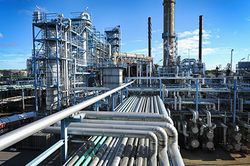# Documentation:CHBE Exam Wiki/Final Exam 2016W/Question 2

CHBE 241
Exam resources wikiChemical and Biological Engineering
Welcome to the CHBE Exam Resources Wiki!

This wiki is intended to host past exams

with fully worked-out hints and solutions
Past Exams
Final Exam 2016W
Midterm Exam 1 2016W
Midterm Exam 2 2016W
Problem Sets
Module 1 - Process Basics
Module 2 - Reactors
Module 3 - Separations 1
Module 4 - Separations 2
Module 5 - Non-reactive Energy Balances
Module 6 - Reactive Energy Balances

A liquid mixture of 70 mol% n-hexane (C6H14) and 30 mol% n-heptane (C7H16) at 40°C is fed at a rate of 100 mol/s into a separating tank, known as a flash drum. In the flash drum, the temperature of the liquid is brought to 80°C and leads to the creation of a vapour phase. The vapour and liquid product streams can be assumed to be in equilibrium and are removed continuously at 80°C. The entire process operates at a uniform 1 atm pressure. Data for this system are provided below:

The Antoine equation is: $log_{10}P^{sat}(mmHg)=A-{\frac {B}{T(^{\circ }C)+C}}$Table 1: Antoine equation coefficients for n-hexane and n-heptane, respectively

Compound A B C
n-hexane 6.87601 1171.17 224.41
n-heptane 6.89677 1264.90 216.54

Table 2: Thermodynamic properties

Compound Cp [J/(mol K)] Ĥvap[kJ/mol)]
n-hexane 150 28.85
n-heptane 180 31.69

Note that Ĥvap can be assumed to be independent of temperature and pressure

## Question 2a [25 points]

Calculate the n-hexane and n-heptane molar compositions in the vapour and liquid product streams as well as the product vapor and liquid stream molar flow rates.

## Question 2b [15 points]

Is energy being transferred to or from the separation tank and what is the amount of energy transferred in kW?

Script error: The function "navbox" does not exist.